Economics
Volume 5, Issue 1, February 2016, Pages: 1-7

The Relative Effectiveness of Monetary and Fiscal Policies on Economic Growth in Bangladesh

Md. Abu Hasan1, Md. Ashraful Islam1, Md. Abul Hasnat1, Md. Abdul Wadud2

2Department of Economics, University of Rajshahi, Rajshahi, Bangladesh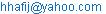(Md. A. Hasan)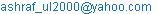(Md. A. Islam)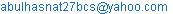(Md. A. Hasnat)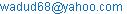(Md. A. Wadud)

Md. Abu Hasan, Md. Ashraful Islam, Md. Abul Hasnat, Md. Abdul Wadud. The Relative Effectiveness of Monetary and Fiscal Policies on Economic Growth in Bangladesh. Economics. Vol. 5, No. 1, 2016, pp. 1-7. doi: 10.11648/j.eco.20160501.11

Abstract: This study explores the relative effectiveness of monetary and fiscal policies on economic growth in Bangladesh for the period from fiscal year 1974 to2015 employing cointegration and Vector Error Correction Model (VECM). We use nominal GDP as a proxy for economic growth, while broad money supply (M2) and reserve money (RM) as proxies for monetary policy. Total government revenue (TR) and total government expenditure (TE) are used as proxies for fiscal policy. The Johansen cointegration tests reveal that monetary policy (M2 and RM) has a greater long run positive impact on economic growth over fiscal policy in Bangladesh. The results of VECM show that there is a weak long run causality running from monetary and fiscal policies to economic growth. VECM also finds that GDP, M2 and TR play a part to adjust any disequilibrium, while TR picks up the disequilibrium rapidly and guides the variables of the system back to equilibrium. VECM Granger causality/block exogeneity Wald test results show that M2 is the leading indicator with respect to economic growth in Bangladesh in the short run. Moreover, economic growth is a leading indicator with respect to fiscal policy in the short run. Thus, we conclude that monetary policy is the more effective channel than fiscal policy to promote economic growth in the short run and long run in Bangladesh.

Keywords: Economic Growth, Monetary Policy, Fiscal Policy, Cointegration, Causality

1. Introduction

The achievement of macroeconomic goals has been a policy precedence of each and every economy. Economic growth has long been regarded as an important macroeconomic goal of economic policy with a considerable frame of research dedicated to explaining how this goal may be accomplished. Fiscal and monetary policies are the main instruments of achieving the macroeconomic goals. Today, monetary and fiscal policies are both commonly accorded outstanding roles in the pursuit of macroeconomic stabilization in developing countries, but the relative efficiency of these policies has been a major debate between the Keynesians and the Monetarists. The debate is rooted in the traditional views of monetarists  and Keynesians . Monetarists rely on monetary policy as they argue that money supply plays a major role in achieving macroeconomic goals, while Keynesians contend that fiscal policy is more important in boosting the economic acivity. The monetarists strongly believe that the central bank must have to increase money supply abruptly owing to accelerate growth in the economy. But concept of liquidity trap disputes that if real interest rate has already reached its minimum level then an increase in money supply may not be able to accelerate output and growth. Then monetary policy will fail to increase investments and restore full employment, while fiscal policy will increase the output through expansion of government expenditure. Considerable volume of empirical work has been carried out by many economists to investigate the relative effectiveness of monetary and fiscal policies and their influence on economic growth [3-11]. There has been contrasting opinions on which of the policies exert greater influence on economic activity. Most researchers, such as, Andersen and Jordan , Ajayi , Ajisafe and Folorunso , Rahman , Ali et al. , Jawaid et al.  and Senbet  find support for the monetarist view, which suggests that monetary policy generally has a greater impact on economic growth and dominates fiscal policy in terms of its impact on real output. Few researchers, such as, Chowdhury, Facklerand McMillin  and Latif and Chowdhury  find that fiscal policy is more effective over monetary policy.

Monetary policy in Bangladesh is conducted by Bangladesh Bank and it targets at attaining a variety of goals, such as, economic growth, price and exchange rate stability, and the development of money and capital markets. Fiscal policy in Bangladesh is conducted by ministry of finance and it basically comprises activities to ensure macroeconomic stability of the country. Fiscal policy in Bangladesh is expansionary which causes large budget deficit; however, the fiscal deficit has been moderated over the years. Over the past 43 years since independence, Bangladesh has increased its real per capita income by more than 130%, cut the poverty rate by 60% and is achieved most of the millennium development goals in spite of frequent natural disasters and political instability . During the recent 15 years, the average economic growth rate has been approached to 6% per annum. Nominal GDP has been increased from Tk. 50 billion to Tk. 15,136 billion from fiscal year 1972-73 to 2014-15. In the same period, total revenue as a percentage of GDP has been risen from 3.43% to 10.8%, while total expenditure as a percentage of GDP has been increased from 11.5% to 18.7% and broad money supply has been increased from about Tk. 12 billion to Tk. 7876 billion [12-13]. Aside from this past progress, Bangladesh is still a lower-middle income country and aspires to be a middle-income country by 2021.

The motivation of this study is generated from the above growth scenario of Bangladesh economy. Thus, the current study finds the answer to the following question: Which macroeconomic policy is relatively effective on economic growth in Bangladesh? This study is expected to add some contributions to the existing literature as there are slightly a few studies performed so far on the subject in context of Bangladesh. The objective of this study is, therefore, to examine the relative effectiveness of monetary and fiscal policy in Bangladesh using the recent econometric modeling techniques of co-integration and Vector Error Correction Model (VECM). The organization of this study is as follows: section 2 focuses research methodology; section 3 presents empirical results and section 4 concludes this study.

2. Methodology

2.1. Data and Data Sources

In this study, we use nominal GDP (proxy for economic growth) as an independent variable, while monetary and fiscal policies are used as explanatory variables. Broad money supply (M2) and reserve money (RM) are used as proxies for monetary policy. Government revenue and government expenditure are used as proxies for fiscal policy. The study uses 42 yearly observations of the variables over a period of fiscal year 1973-74 to 2014-15. Data are collected from various issues of the monthly economic trends published by Bangladesh Bank, Bangladesh Economic Review published by Ministry of Finance and Sixth Five Year Plan published by Ministry of Planning [12-14].

2.2. Research Methods

All data series are transformed to natural logarithms. The rationale for considering log is that taking the natural logarithm of a series effectively linearizes the exponential trend (if any) in the time series data as the log function is the inverse of an exponential function . Modern econometrics methods are applied in this study to obtain the answer of the research question. Descriptive statistics are used to provide a general understanding of the empirical features of the variables incorporated in this study. Unit root tests and cointegration analysis are employed to test the stationarity and multiple long-run relationship respectively. Vector Error Correction Model (VECM) is operated to test the short and long run causality, and reconcile short run to long run behavior of the variables.

2.2.1. Unit Root Test

The cointegration analysis requires the variables which must be integrated in the same order. Examinations that may verify the order of integration are referred to as unit root tests. Three regression models intercept and trend, intercept, and none are used in this study to test for unit root. Two extensively used unit root test, namely Augmented Dickey Fuller (ADF) and Phillips-Peron (PP) test are employed to examine the stationarity of the time series. The ADF test is performed using the following equation: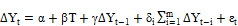(1)

where, α is a interecpt (constant), β is the coefficient of time trend T, γ and δ are the parameters where, γ = ρ-1, ∆Y is the first difference of Y series, m is the number of lagged first differenced term, and ε is the error term.

Phillips and Perron  test is performed using the following equation: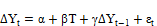(2)

where, α is a constant, β is the coefficient of time trend T, γ is the parameter and ε is the error term.

2.2.2. The Johansen Cointegration Analysis

Johansen and Juselius  cointegration approach based the on VAR model is applied to examine the long run relationship that may exist among representative variables. Five different criteria are used to determine the lag lengths used in the VAR. Residual serial correlation LM test is performed to select appropriate lag lengths for the VAR. The Johansen and Juselius (JJ) approach can be expressed mathematically as: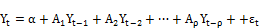(3)

where Yt is a vector containing n variables of I(1) at time t, α is an (n× 1) vector of constants, Aρ is an (n× n) matrix of coefficients, ρ is the maximum lag included in the model and εt is an (n× 1) vector of error terms.

Equation (3) can be written in the form of the error correction model assuming cointegration of order ρ as in :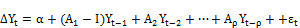(4)

or in a final broad form as: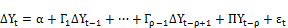(5)

where, Гi =(A1 + A2 + ...+ Aρ −1 −I) represents the dynamics of the model in the short run. In (5), 𝛱=(A1 +A2 + ...+ Aρ I) represents the long run relationship among the variables included in the vector 𝑌𝑡, and I is the identity vector. The key idea of the JJ approach is to determine the rank of the matrix 𝛱, which represents the number of independent cointegration vectors.

Johansen  suggests two test statistics named trace and eigenvalue test statistic for estimating the number of cointegrating vectors or equations. The trace and maximum eigenvalue test are as follows: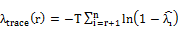(6)

and(7)

where, T is the sample size andis the eigenvalues.

2.2.3. Vector Error Correction Model (VECM)

Vector Error Correction Model (VECM) is implemented to investigate the long run causality and short run to long run dynamic adjustment of a system of cointegrated variables. Short run causality between DSI and macroeconomic variables is determined using VECM Granger causality/block exogeneity Wald tests.

Equation (4) can be written as a VECM as: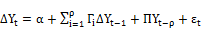(8)

where, Γi=A1+A2+A3 +………. +Aρ-1 I represents the dynamics of the model in the short-run and П = A1+A2+A3 +………. +Aρ–I is the long-run relationship among the variables included in the vector Yt and I is the identity vector. ∆Yt is an nx1 vector of variables and α is an (nx1) vector of constants. Π is the errorcorrection mechanism, which has two components: Π=µβ′ where µ is an (nx1) column vector representing the speed of the short run adjustment to the long-run equilibrium, and β′ is a (1xn) cointegrating vector with the matrix of long run coefficients. Γ is an (nxn) matrix representing the coefficients of the short run dynamics. Finally, εt is an (nx1) vector of white noise error terms, and ρ is the order of the autoregression.

3. Empirical Results

3.1. Descriptive Statistics

Table 1 presents the summary statistics including mean, minimum and maximum values, standard deviation, kurtosis, skewness, and Jarque-Bera test for data under consideration in their log levels. We can observe that standard deviations of M2 and RM are higher than the other variables. It means monetary policy variables are more volatile compared to other variables. The negative skewness values of all the variables indicate that the variables are skewed to the left meaning that the left tails are longer. The kurtosis of macroeconomic variables are less than 3, which indicate that the distributions are platykurtic. The calculated Jarque-Bera statistics and P-values in Table 1 are used to test the null hypothesis for normal distribution (H0: Yearly distribution is normally distributed). The P-values reveal that the null hypothesis is accepted for all the variables meaning that they are normally distributed.

Table 1. Statistical features of the variables in log level.

 Statistics GDP M2 RM TR TE Mean 7.178074 5.835672 4.381280 5.208688 4.678793 Median 7.270284 5.971267 4.683509 5.352753 4.901021 Maximum 9.624831 8.971593 7.303052 7.948185 7.399207 Minimum 4.330733 2.521319 1.131402 2.038099 1.076230 Std.Dev. 1.424386 1.905353 1.804023 1.509650 1.643833 Skewness -0.231554 -0.147087 -0.276415 -0.152879 -0.254478 Kurtosis 2.211715 1.993227 2.106765 2.258885 2.183098 Jarque-Bera 1.462758 1.925226 1.931108 1.124792 1.621139 Probability 0.481245 0.381894 0.380772 0.569842 0.444605 Observations 42 42 42 42 42

3.2. Unit Root Test Results

Considering the results of ADF and PP tests (Table 2), it is clearly evident that the null hypothesis of a unit root at the level are accepted in all cases for GDP, M2 and RM as test statistics are lower than the critical values. Considering the intercept term for the TR and TE series in PP test, we can conclude that TR and TE also have unit root in level. Therefore, we conclude that all series are nonstationary in levels. Results from the ADF and PP tests provide that all series are stationary in first differences with 1% significance level. So, all the individual series are found to be integrated of order one, i.e., I(1). As a result, the following analysis is conducted under the assumption that all variables are stationary in first differences.

Table 2. ADF and PP unit root test results of the variables.

 GDP ∆GDP M2 ∆M2 RM ∆RM TR ∆TR TE ∆TE ADF Intercept -.45 -8.11* -.63 -4.45* -1.04 -4.45* -3.1** -5.76* -1.64 -6.17* (.89) (.00) (.85) (.00) (.73) (.00) (.03) (.00) (.45) (.00) Trend & Intercept -1.41 -7.92* -3.12 -4.69* -1.39 -4.44* -7.05* -5.69* -3.9** -6.09* (.84) (.00) (.11) (.00) (.85) (.00) (.00) (.00) (.01) (.00) PP Intercept -1.57 -7.78* -.82 -4.49* -.93 -4.45* -2.78 -6.49* -1.57 -6.26* (.49) (.00) (.80) (.00) (.77) (.00) (.07) (.00) (.48) (.00) Trend & Intercept -2.78 -7.62* -1.45* -4.68* -1.64 -4.44* -7.05* -6.18* 3.8** -6.15* (.21) (.00) (.83) (.00) (.76) (.00) (.00) (.00) (.02) (.00)

Notes: First bracket shows P-values. * and **indicate stationary at 1% and 5% levels respectively using MacKinnon (1996) critical and P -values.

3.3. Selection of Optimal Lag Lengths for the VAR

The number of cointegrating vectors generated by Johansen approach may be sensitive to the number of lags in the VAR model . Henceforth, in this study, we use and check five different criteria namely, Likelihood Ratio (LR), Final Prediction Error (FPE), Akaike Information Criteria (AIC), Schwarz Information Criteria (SIC) and Hannan-Quinn Information Criteria (HQ) to determine the optimum lag lengths of the VAR model. Results for each criterion with a maximum of 3 lags exhibit that AIC, sequential modified LR and FPE criteriastand in favor of 2 lags, while SIC and HQ criteria suggest for only 1 lag (Table 3). The presence of residual serial correlation makes the result less efficient. Thus, we proceed to conduct LM tests for each suggested lags up to maximum 3 lags. Results of the Lagrange Multiplier (LM) test strongly reveal the presence of serial correlation in the estimated residuals generated from VAR (1) model up to 1 year (Table 4). Using 2 lags suggested by AIC, sequential modified LR and FPE criteria produces no autocorrelation in the VAR model for up to 3 years. So, we accept VAR (2) model for cointegrating analysis.

Table 3. Optimal lag lengths of the VAR model.

 Lag LogL LR FPE AIC SIC HQ 0 67.21444 NA 2.83e-08 -3.190484 -2.977207 -3.113962 1 283.6363 366.2523 1.56e-12 -13.00699 -11.72732* -12.54786* 2 313.7535 43.24532* 1.29e-12* -13.26941* -10.92336 -12.42767 3 334.6466 24.64310 1.90e-12 -13.05880 -9.646366 -11.83445

Note: * indicates lag order selected by the criterion.

Table 4. Residual serial correlation LM tests for the VAR model.

 Lags 2 Lags 1 Lag LM-Stat P-Values LM-Stat P-Values 1 25.26845 .44 45.54878 .00 2 30.84073 .19 28.63178 .27 3 29.97443 .22 16.91736 .88

Note: Probs from chi-square with 25 df.

3.4. Results of Long Run Relationship Based on Johansen Cointegration Test

We progress the Johansen cointegration test using the default option of the EViews 8.1, which assumes linear trend in the VAR and the cointegrating relationship only has an intercept. Table 5 shows that the null hypothesis of there are at most 1 cointegrating vector can be rejected since the λtrace statistics of 78.96 is greater than its critical value  of 69.81 at the 1% level of significance. Hence, the trace test indicates 1 cointegrating equation at the 1% level. In contrast, the null hypothesis of there is no cointegrating vector can be rejected since the λmax statistics of 39.28 is greater than its critical value of 33.87 at the 5% level of significance. Thus, the maximum eigenvalue test suggests for 1 cointegrating vector at the 5% level of significance.

Table 5. Johansen-Juselius cointegration test results.

 Unrestricted Cointegration Rank Test (Trace) Unrestricted Cointegration Rank Test (Maximum Eigenvalue) H0 H1 Trace Statistic 5% Critical Value P *** H0 H1 Max-Eigen Statistics 5% Critical Value P *** r = 0 r > 0 78.96206** 69.81889 .00 r = 0 r = 1 39.28483** 33.87687 .01 r≤1 r > 1 39.67723 47.85613 .23 r = 1 r = 2 22.08438 27.58434 .21 r≤2 r > 2 17.59285 29.79707 .59 r = 2 r = 3 10.67798 21.13162 .67 r≤3 r > 3 6.914873 15.49471 .58 r = 3 r = 4 6.581274 14.26460 .53 r≤4 r > 4 0.333599 3.841466 .56 r = 4 r = 5 0.333599 3.841466 .56

Notes: r indicates the number of cointegrating relationships. * and ** denote rejection of the null hypothesis at the 1% and 5% levels respectively. *** indicates MacKinnon-Haug-Michelis (1999) p-values.

It can be taken from the Johansen-Juselius cointegration test that there are at least one cointegrating vectors in the system indicating that there are at least one long run relationship among the variables. Thus, the cointegrating vector is normalized on GDP. The normalized cointegrating coefficient gives the long run relationship between GDP and explanatory variables. The signs of coefficients are reversed because of the normalization process. The estimation of the equation by cointegration gives the following long run equation:

GDP = 2.51 + .42 M2 + .02 RM–.25 TR + .64 TE     (9)

(.14)        (.08)       (.14)      (.17)

[-2.88]      [-.23]      [1.81]    [-3.85]

Note: Standard Erros in parentheses and t-statistics in square brackets.

In the long run, total revenue have a negative relationship with economic growth (proxied by GDP). On the other hand, broad money supply and total expenditure have a significant long run positive relationship with GDP. Reserve money is also positively related with GDP; however, the relationship is not significant. The result is implying that a 1% increase in TR contributes to 0.25% decrease in economic growth in Bangladesh. Besides, a 1% increase in M2, TE and RM contributes to 0.42%, 0.64% and 0.02% increase in economic growth respectively.

3.5. Results of Long Run Causality and Speed of Adjustment Based on VECM

The study reduces the lag length by -1 in the lag length as we go from the VAR to the VECM. Table 6 presents VEC(1) estimates for the variables. The result shows that the coefficient of error correction term on the regression with first difference GDP is negative and also significant at 10% level based on t-statistics and P-value. It indicates that there is a long run causality running from the explanatory variables (M2, RM, TR and TE) to the dependent variable (GDP). The results of the estimated multivariate VECM clearly indicate that the coefficients of error correction terms of the first differenced M2 and TR equations are negative and statistically significant at the 5% and 1% level of significance respectively. It suggests that the long run causality is also directing from GDP to M2 and GDP to TR. The error correction term of first differenced GDP is -0.25, which implies that GDP requires about four years to converge into equilibrium after being shocked. Thus, only 25% of the last year’s disequilibrium is corrected this year by changes in GDP. The VEC estimates also reveal that the error correction terms of first differenced M2, RM and TR are significant; however, the coefficient of error correction term of the first differenced RM equation is not correctly signed. So, adjustment of the disequilibrium of M2 and TR towards a long run equilibrium state takes about more than 5 years and less than 2 years respectively. Above and beyond, the coefficient of error correction terms of TE equationis correctly signed but not significant. Thus, we can conclude that GDP, M2 and TR contribute to adjust any disequilibrium, while TR picks up the disequilibrium quickly and guides the variables of the system back to equilibrium.

Table 6. Vector error correction estimates.

 Error Correction D(GDP) D(M2) D(RM) D(TR) D(TE) Coint. Equation -0.251738*** -0.194267** 0.606905* -0.563745* -0.288800 Standard Errors 0.15076 0.08770 0.22518 0.13032 0.20078 t-statistics -1.66980 -2.21511 2.69520 -4.32576 -1.43841 P-value .09 .02 .00 .00 .15

Note: *,**and *** denote significance at 1%, 5% and 10% level respectively.

3.6. Results of Short Run Causality Based on VECM Granger Causality/Block Exogeneity Wald Tests

Short run causality between GDP and explanatory variables is determined with a test on the individual and joint significance of the lagged explanatory variables using VECM Granger causality/block exogeneity Wald tests. Under this system, an endogenous variable can be treated as exogenous. This test detects whether the lags of one excluded variable can Granger cause the dependent variable in the VAR system using the Chi-square (Wald) statistics. Results are reported in Table 7. In the case, where GDP is the dependent variable and M2, RM, TR and TE are the joint excluded variables, the Chi-square probability value of the excluded variables is 0.00 (which is less than 1%). This means that there is a short run Granger causality running jointly from M2, RM, TR and TE to GDP. In GDP equation, the Chi-square statistics of D(M2) is significant at1% level, while the Chi-square probability value of D(GDP) in M2 equation is not significant. This means that there is a significant unidirectional short run Granger causality running from M2 to GDP in Bangladesh. There is unidirectional short run causality running from GDP to fiscal policy (TR and TE) at 1% level of significance.

Table 7. Results of the VECM granger causality/block exogeneity wald tests.

 Dependent Variable Excluded Chi-Square Statistics Degrees of Freedom P-value D(GDP) D(M2) 7.930570* 1 .00 D(RM) 4.36E-05 1 .99 D(TR) 1.744559 1 .18 D(TE) 0.029567 1 .86 All 16.99870* 4 .00 D(M2) D(GDP) 0.266382 1 .60 D(RM) D(GDP) 0.073208 1 .78 D(TR) D(GDP) 47.93938* 1 .00 D(TE) D(GDP) 7.405980* 1 .00

Note: *denotes significance at 1% level.

In order to determine the robustness of the model, diagostic ckecking of the estimated model has been carried out in terms of conventional multivariate residual-based tests for autocorrelation and heteroskedasticity. At 1% level of significance, the multivariate Lagrange Multiplier (LM) test for autocorrelation indicates the absence of autocorrelation at all lags, and White’s Chi-square test for heteroskedasticity indicates the absence of heteroskedasticity.

4. Conclusion

This study uses fiscal yearwise data from 1973-74 to 2014-15 of monetary policy (broad money supply and reserve money) and fiscal policy (total revenue and total expenditure) variables and relates it to real economic activity (GDP) to investigate there relative effectiveness of monetary and fiscal policies on economic growth in Bangladesh. The Johansen cointegration tests reveal that monetary policy (broad money supply and reserve money) have a long run positive relationship with economic growth. On the other hand, total expenditure has a negative long run relationship, while total expenditure has a positive long run relationship with economic growth in Bangladesh. Thus, we can comment that monetary policy is the more effective instrument to promote economic growth in the long run in Bangladesh. The results of VECM indicate that there is a weak long run causality running from monetary and fiscal policies to economic growth. The coefficients of error correction terms of the first differenced GDP, M2 and TR reveal that GDP, M2 and TR contribute to adjust any disequilibrium, while TR picks up the disequilibrium quickly (less than two years) and guides the variables of the system back to equilibrium. VECM Granger causality/block exogeneity Wald test results show that there is a significant short run Granger causality running jointly from monetary and fiscal policies to economic growth. The test also reveals that there is a short run Granger causality running from M2 to GDP at 1% level of significance. Thus, individually M2 is the leading indicator with respect to economic growth in Bangladesh in the short run. Moreover, economic growth is a leading indicator with respect to fiscal policy in the short run. Thus, we can comment that monetary policy is also the more effective instrument to promote economic growth in the short run in Bangladesh.

We find support for the monetarist observation and empirical studies as in [3,4,6,10], which advocates that monetary policy largely has a superior effect on economic growth over fiscal policy. Future researchers can investigate there relative effectiveness of monetary and fiscal policies on economic growth including data of other monetary and fiscal policies variables. Finally, we conclde that sound coordination of monetary policy is necessitated in Bangladesh as the economic growth of Bangladesh is motivated by monetary policy factors in the short run and long run.

References

1. Friedman, M.& Schwartz, A. (1963). A monetary history of the United States, 1867-1960. Princeton: Princeton University Press.
2. Ando, A.& Modigliani, F. (1965). The relative stability of monetary velocity and the multiplier. American Economic Review, 3: 693-728.
3. Andersen, L. C. & Jordan, J. L. (1968). Monetary and fiscal actions: a test of their relative importance in economic stabilization. Federal Reserve Bank of St. Louis Review, 52: 7-27.
4. Ajayi, S.I. (1974). An econometric case study of the relative importance of monetary and fiscal policy in Nigeria. The Bangladesh Economic Review, 2(2): 559-576.
5. Latif, E.& Chowdhury, M. H. (1998), Relative effectiveness of monetary and fiscal policy, Bank Parikrama, 23: 1&2: 97-105.
6. Ajisafe, R. A. & Folorunso, B. A. (2002).The relative effectiveness of fiscal and monetary policy in macroeconomic management in Nigeria. The African Economic and Business Review, 3(1): 23-40.
7. Rahman, M. H. (2005). Relative effectiveness of monetary and fiscal policies on output growth in Bangladesh: a VAR approach, Bangladesh Bank Working Paper Series: WP 0601.
8. Ali, S. Irum, S. & Ali, A. (2008). Whether fiscal stance or monetary policy is effective for economic growth in case of South Asian countries. The Pakistan Development Review, 47(4): 791-799.
9. Jawaid, S. T. Arif, I.& Naeemullah, S. M. (2010). Comparative analysis of monetary and fiscal policy: a case study of Pakistan. NICE Research Journal, 3: 58-67.
10. Senbet, D. (2011). The relative impact of fiscal versus monetary actions on output: a Vector Autoregressive (VAR) approach. Business and Economic Journal, 25: 1-11.Retrieved from http://astonjournals.com/manuscripts/Vol2011/BEJ25Vol2011.pdf.
11. Chowdhury, A. Fackler, J. S.& McMillin, W. D. (1986). Monetary policy, fiscal policy and investment spending: An empirical analysis. Southern Economic Journal, 52(3): 794-805.
12. Ministry of Planning, People’s Republic of Bangladesh, (2011). Sixth Five Year Plan FY2011-FY2015: Accelerating growth and reducing poverty, viewed 10 November 2014, http://www.plancomm.gov.bd/sixth-five-year-plan/
13. Bangladesh Bank. (2015). Monthly Economic Trends. https://www.bb.org.bd/econdata/time_series_data1972-2015.xls
14. Ministry of Finance. (2013). People’s Republic of Bangladesh. Bangladesh Economic Review 2013.
15. Asteriou, D.&Price, S. (2007). Applied econometrics: a modern approach, New York: Palgrave Macmillan Inc.
16. Phillips, P. C. B. & Perron, P. (1988). Testing for a unit root in time series regression. Biometrika, 75(2): 335-346.
17. Johansen, S.& Juselius, K. (1990). Maximum likelihood estimation and inference on cointegration with applications to the demand for money. Oxford Bulletin of Economics and Statistics, 52(2): 169-210.
18. Enders, W. (2004). Applied econometric time series. 2nded., John Wiley & Sons Inc, New York.
19. Johansen, S. (1988). Statistical analysis of cointegrating vectors. Journal of Economic Dynamics and Control, 12: 231-54.

 Contents 1. 2. 2.1. 2.2. 3. 3.1. 3.2. 3.3. 3.4. 3.5. 3.6. 4.
Article ToolsAbstractPDF(255K)